Publicité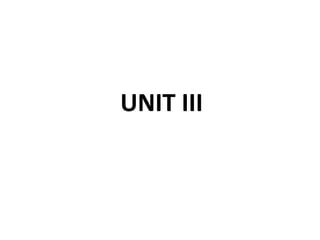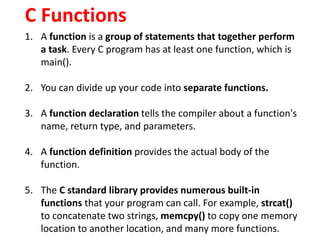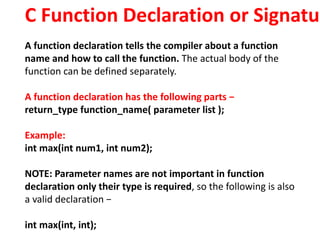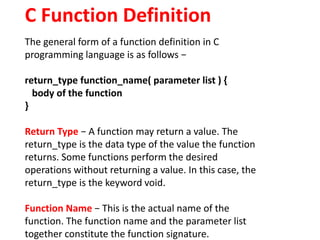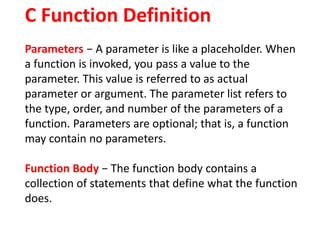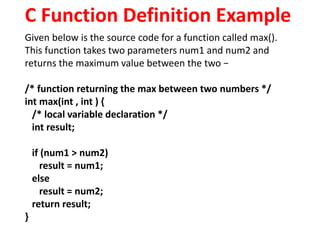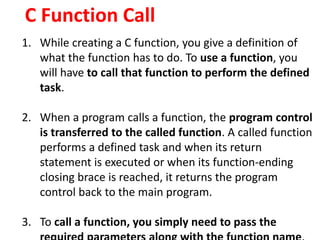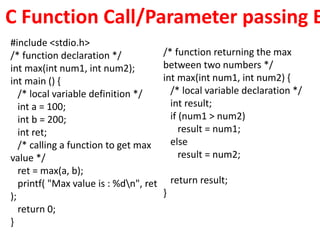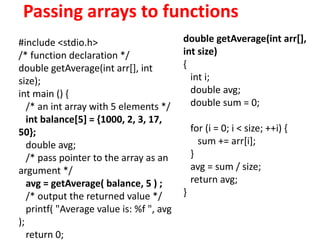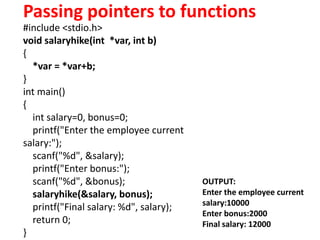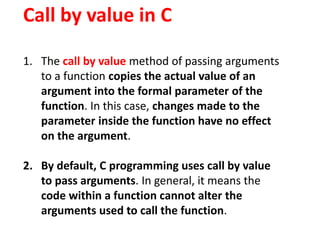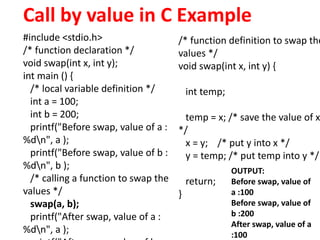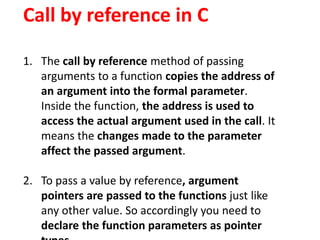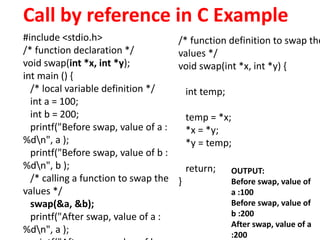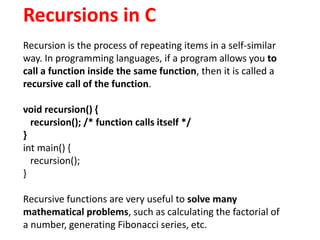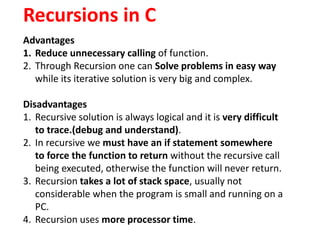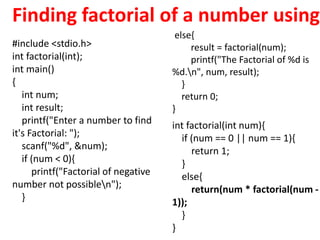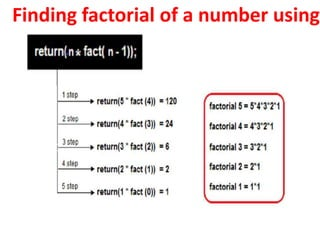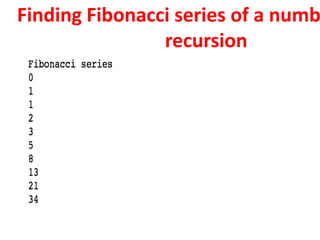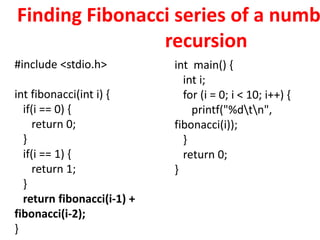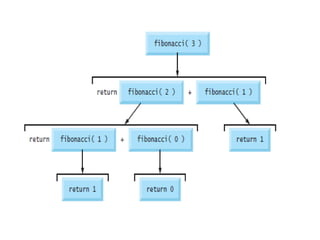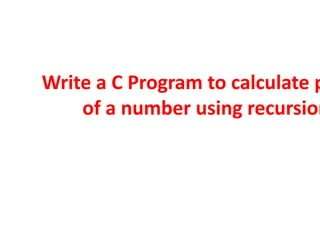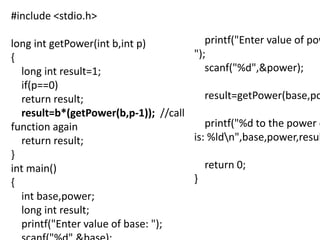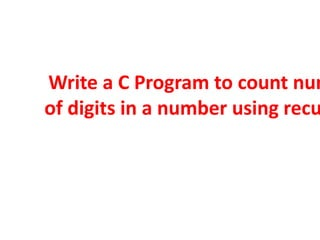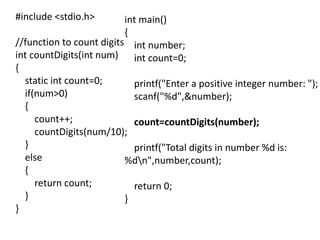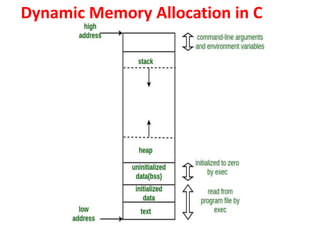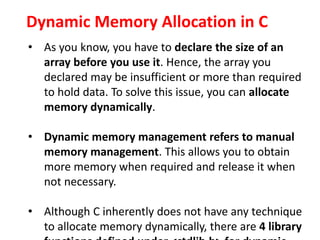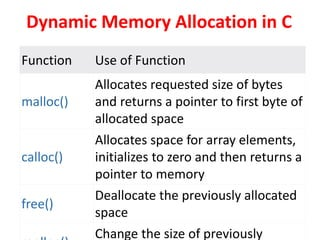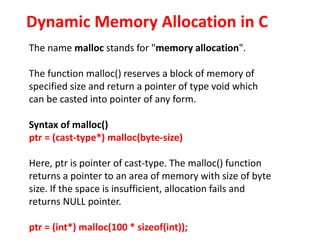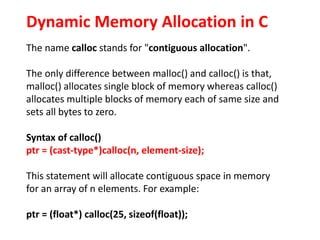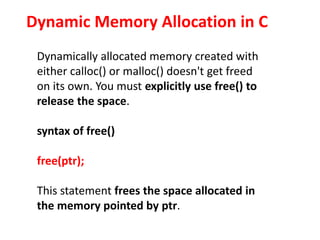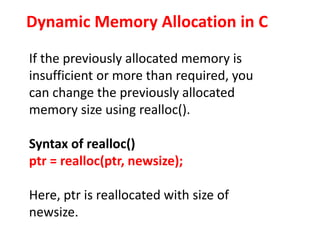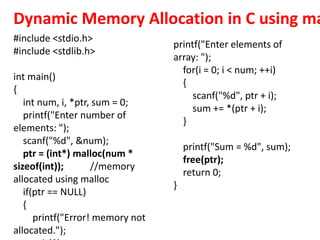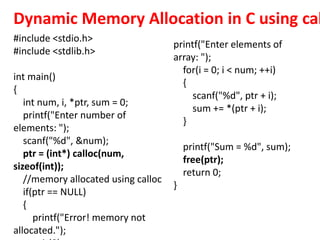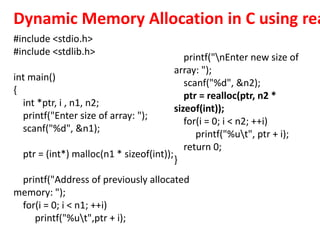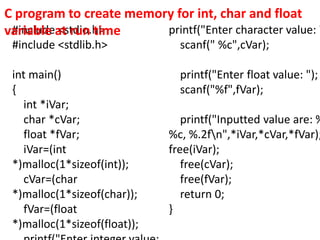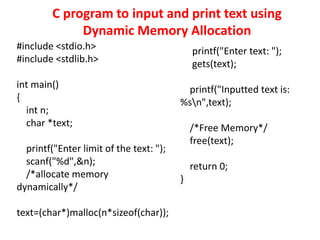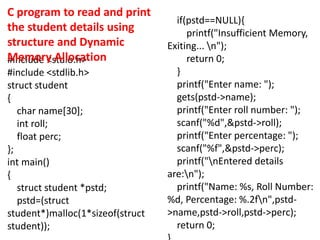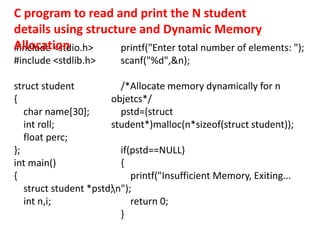1 sur 39
Publicité

### UNIT3.pptx

1. UNIT III
2. C Functions 1. A function is a group of statements that together perform a task. Every C program has at least one function, which is main(). 2. You can divide up your code into separate functions. 3. A function declaration tells the compiler about a function's name, return type, and parameters. 4. A function definition provides the actual body of the function. 5. The C standard library provides numerous built-in functions that your program can call. For example, strcat() to concatenate two strings, memcpy() to copy one memory location to another location, and many more functions.
3. C Function Declaration or Signatur A function declaration tells the compiler about a function name and how to call the function. The actual body of the function can be defined separately. A function declaration has the following parts − return_type function_name( parameter list ); Example: int max(int num1, int num2); NOTE: Parameter names are not important in function declaration only their type is required, so the following is also a valid declaration − int max(int, int);
4. C Function Definition The general form of a function definition in C programming language is as follows − return_type function_name( parameter list ) { body of the function } Return Type − A function may return a value. The return_type is the data type of the value the function returns. Some functions perform the desired operations without returning a value. In this case, the return_type is the keyword void. Function Name − This is the actual name of the function. The function name and the parameter list together constitute the function signature.
5. C Function Definition Parameters − A parameter is like a placeholder. When a function is invoked, you pass a value to the parameter. This value is referred to as actual parameter or argument. The parameter list refers to the type, order, and number of the parameters of a function. Parameters are optional; that is, a function may contain no parameters. Function Body − The function body contains a collection of statements that define what the function does.
6. C Function Definition Example Given below is the source code for a function called max(). This function takes two parameters num1 and num2 and returns the maximum value between the two − /* function returning the max between two numbers */ int max(int , int ) { /* local variable declaration */ int result; if (num1 > num2) result = num1; else result = num2; return result; }
7. C Function Call 1. While creating a C function, you give a definition of what the function has to do. To use a function, you will have to call that function to perform the defined task. 2. When a program calls a function, the program control is transferred to the called function. A called function performs a defined task and when its return statement is executed or when its function-ending closing brace is reached, it returns the program control back to the main program. 3. To call a function, you simply need to pass the
8. C Function Call/Parameter passing E #include <stdio.h> /* function declaration */ int max(int num1, int num2); int main () { /* local variable definition */ int a = 100; int b = 200; int ret; /* calling a function to get max value */ ret = max(a, b); printf( "Max value is : %dn", ret ); return 0; } /* function returning the max between two numbers */ int max(int num1, int num2) { /* local variable declaration */ int result; if (num1 > num2) result = num1; else result = num2; return result; }
9. Passing arrays to functions #include <stdio.h> /* function declaration */ double getAverage(int arr[], int size); int main () { /* an int array with 5 elements */ int balance = {1000, 2, 3, 17, 50}; double avg; /* pass pointer to the array as an argument */ avg = getAverage( balance, 5 ) ; /* output the returned value */ printf( "Average value is: %f ", avg ); return 0; double getAverage(int arr[], int size) { int i; double avg; double sum = 0; for (i = 0; i < size; ++i) { sum += arr[i]; } avg = sum / size; return avg; }
10. Passing pointers to functions #include <stdio.h> void salaryhike(int *var, int b) { *var = *var+b; } int main() { int salary=0, bonus=0; printf("Enter the employee current salary:"); scanf("%d", &salary); printf("Enter bonus:"); scanf("%d", &bonus); salaryhike(&salary, bonus); printf("Final salary: %d", salary); return 0; } OUTPUT: Enter the employee current salary:10000 Enter bonus:2000 Final salary: 12000
11. Call by value in C 1. The call by value method of passing arguments to a function copies the actual value of an argument into the formal parameter of the function. In this case, changes made to the parameter inside the function have no effect on the argument. 2. By default, C programming uses call by value to pass arguments. In general, it means the code within a function cannot alter the arguments used to call the function.
12. Call by value in C Example #include <stdio.h> /* function declaration */ void swap(int x, int y); int main () { /* local variable definition */ int a = 100; int b = 200; printf("Before swap, value of a : %dn", a ); printf("Before swap, value of b : %dn", b ); /* calling a function to swap the values */ swap(a, b); printf("After swap, value of a : %dn", a ); /* function definition to swap the values */ void swap(int x, int y) { int temp; temp = x; /* save the value of x */ x = y; /* put y into x */ y = temp; /* put temp into y */ return; } OUTPUT: Before swap, value of a :100 Before swap, value of b :200 After swap, value of a :100
13. Call by reference in C 1. The call by reference method of passing arguments to a function copies the address of an argument into the formal parameter. Inside the function, the address is used to access the actual argument used in the call. It means the changes made to the parameter affect the passed argument. 2. To pass a value by reference, argument pointers are passed to the functions just like any other value. So accordingly you need to declare the function parameters as pointer
14. Call by reference in C Example #include <stdio.h> /* function declaration */ void swap(int *x, int *y); int main () { /* local variable definition */ int a = 100; int b = 200; printf("Before swap, value of a : %dn", a ); printf("Before swap, value of b : %dn", b ); /* calling a function to swap the values */ swap(&a, &b); printf("After swap, value of a : %dn", a ); /* function definition to swap the values */ void swap(int *x, int *y) { int temp; temp = *x; *x = *y; *y = temp; return; } OUTPUT: Before swap, value of a :100 Before swap, value of b :200 After swap, value of a :200
15. Recursions in C Recursion is the process of repeating items in a self-similar way. In programming languages, if a program allows you to call a function inside the same function, then it is called a recursive call of the function. void recursion() { recursion(); /* function calls itself */ } int main() { recursion(); } Recursive functions are very useful to solve many mathematical problems, such as calculating the factorial of a number, generating Fibonacci series, etc.
16. Recursions in C Advantages 1. Reduce unnecessary calling of function. 2. Through Recursion one can Solve problems in easy way while its iterative solution is very big and complex. Disadvantages 1. Recursive solution is always logical and it is very difficult to trace.(debug and understand). 2. In recursive we must have an if statement somewhere to force the function to return without the recursive call being executed, otherwise the function will never return. 3. Recursion takes a lot of stack space, usually not considerable when the program is small and running on a PC. 4. Recursion uses more processor time.
17. #include <stdio.h> int factorial(int); int main() { int num; int result; printf("Enter a number to find it's Factorial: "); scanf("%d", &num); if (num < 0){ printf("Factorial of negative number not possiblen"); } int factorial(int num){ if (num == 0 || num == 1){ return 1; } else{ return(num * factorial(num - 1)); } } else{ result = factorial(num); printf("The Factorial of %d is %d.n", num, result); } return 0; } Finding factorial of a number using
18. Finding factorial of a number using
19. Finding Fibonacci series of a numb recursion
20. Finding Fibonacci series of a numb recursion #include <stdio.h> int fibonacci(int i) { if(i == 0) { return 0; } if(i == 1) { return 1; } return fibonacci(i-1) + fibonacci(i-2); } int main() { int i; for (i = 0; i < 10; i++) { printf("%dtn", fibonacci(i)); } return 0; }
21. Write a C Program to calculate p of a number using recursion
22. #include <stdio.h> long int getPower(int b,int p) { long int result=1; if(p==0) return result; result=b*(getPower(b,p-1)); //call function again return result; } int main() { int base,power; long int result; printf("Enter value of base: "); printf("Enter value of pow "); scanf("%d",&power); result=getPower(base,po printf("%d to the power o is: %ldn",base,power,resul return 0; }
23. Write a C Program to count num of digits in a number using recu
24. #include <stdio.h> //function to count digits int countDigits(int num) { static int count=0; if(num>0) { count++; countDigits(num/10); } else { return count; } } int main() { int number; int count=0; printf("Enter a positive integer number: "); scanf("%d",&number); count=countDigits(number); printf("Total digits in number %d is: %dn",number,count); return 0; }
25. Dynamic Memory Allocation in C
26. Dynamic Memory Allocation in C • As you know, you have to declare the size of an array before you use it. Hence, the array you declared may be insufficient or more than required to hold data. To solve this issue, you can allocate memory dynamically. • Dynamic memory management refers to manual memory management. This allows you to obtain more memory when required and release it when not necessary. • Although C inherently does not have any technique to allocate memory dynamically, there are 4 library
27. Dynamic Memory Allocation in C Function Use of Function malloc() Allocates requested size of bytes and returns a pointer to first byte of allocated space calloc() Allocates space for array elements, initializes to zero and then returns a pointer to memory free() Deallocate the previously allocated space Change the size of previously
28. The name malloc stands for "memory allocation". The function malloc() reserves a block of memory of specified size and return a pointer of type void which can be casted into pointer of any form. Syntax of malloc() ptr = (cast-type*) malloc(byte-size) Here, ptr is pointer of cast-type. The malloc() function returns a pointer to an area of memory with size of byte size. If the space is insufficient, allocation fails and returns NULL pointer. ptr = (int*) malloc(100 * sizeof(int)); Dynamic Memory Allocation in C
29. Dynamic Memory Allocation in C The name calloc stands for "contiguous allocation". The only difference between malloc() and calloc() is that, malloc() allocates single block of memory whereas calloc() allocates multiple blocks of memory each of same size and sets all bytes to zero. Syntax of calloc() ptr = (cast-type*)calloc(n, element-size); This statement will allocate contiguous space in memory for an array of n elements. For example: ptr = (float*) calloc(25, sizeof(float));
30. Dynamic Memory Allocation in C Dynamically allocated memory created with either calloc() or malloc() doesn't get freed on its own. You must explicitly use free() to release the space. syntax of free() free(ptr); This statement frees the space allocated in the memory pointed by ptr.
31. Dynamic Memory Allocation in C If the previously allocated memory is insufficient or more than required, you can change the previously allocated memory size using realloc(). Syntax of realloc() ptr = realloc(ptr, newsize); Here, ptr is reallocated with size of newsize.
32. #include <stdio.h> #include <stdlib.h> int main() { int num, i, *ptr, sum = 0; printf("Enter number of elements: "); scanf("%d", &num); ptr = (int*) malloc(num * sizeof(int)); //memory allocated using malloc if(ptr == NULL) { printf("Error! memory not allocated."); printf("Enter elements of array: "); for(i = 0; i < num; ++i) { scanf("%d", ptr + i); sum += *(ptr + i); } printf("Sum = %d", sum); free(ptr); return 0; } Dynamic Memory Allocation in C using ma
33. #include <stdio.h> #include <stdlib.h> int main() { int num, i, *ptr, sum = 0; printf("Enter number of elements: "); scanf("%d", &num); ptr = (int*) calloc(num, sizeof(int)); //memory allocated using calloc if(ptr == NULL) { printf("Error! memory not allocated."); printf("Enter elements of array: "); for(i = 0; i < num; ++i) { scanf("%d", ptr + i); sum += *(ptr + i); } printf("Sum = %d", sum); free(ptr); return 0; } Dynamic Memory Allocation in C using cal
34. Dynamic Memory Allocation in C using rea #include <stdio.h> #include <stdlib.h> int main() { int *ptr, i , n1, n2; printf("Enter size of array: "); scanf("%d", &n1); ptr = (int*) malloc(n1 * sizeof(int)); printf("Address of previously allocated memory: "); for(i = 0; i < n1; ++i) printf("%ut",ptr + i); printf("nEnter new size of array: "); scanf("%d", &n2); ptr = realloc(ptr, n2 * sizeof(int)); for(i = 0; i < n2; ++i) printf("%ut", ptr + i); return 0; }
35. #include <stdio.h> #include <stdlib.h> int main() { int *iVar; char *cVar; float *fVar; iVar=(int *)malloc(1*sizeof(int)); cVar=(char *)malloc(1*sizeof(char)); fVar=(float *)malloc(1*sizeof(float)); printf("Enter character value: " scanf(" %c",cVar); printf("Enter float value: "); scanf("%f",fVar); printf("Inputted value are: % %c, %.2fn",*iVar,*cVar,*fVar); free(iVar); free(cVar); free(fVar); return 0; } C program to create memory for int, char and float variable at run time
36. C program to input and print text using Dynamic Memory Allocation #include <stdio.h> #include <stdlib.h> int main() { int n; char *text; printf("Enter limit of the text: "); scanf("%d",&n); /*allocate memory dynamically*/ text=(char*)malloc(n*sizeof(char)); printf("Enter text: "); gets(text); printf("Inputted text is: %sn",text); /*Free Memory*/ free(text); return 0; }
37. #include <stdio.h> #include <stdlib.h> struct student { char name; int roll; float perc; }; int main() { struct student *pstd; pstd=(struct student*)malloc(1*sizeof(struct student)); if(pstd==NULL){ printf("Insufficient Memory, Exiting... n"); return 0; } printf("Enter name: "); gets(pstd->name); printf("Enter roll number: "); scanf("%d",&pstd->roll); printf("Enter percentage: "); scanf("%f",&pstd->perc); printf("nEntered details are:n"); printf("Name: %s, Roll Number: %d, Percentage: %.2fn",pstd- >name,pstd->roll,pstd->perc); return 0; C program to read and print the student details using structure and Dynamic Memory Allocation
38. #include <stdio.h> #include <stdlib.h> struct student { char name; int roll; float perc; }; int main() { struct student *pstd; int n,i; printf("Enter total number of elements: "); scanf("%d",&n); /*Allocate memory dynamically for n objetcs*/ pstd=(struct student*)malloc(n*sizeof(struct student)); if(pstd==NULL) { printf("Insufficient Memory, Exiting... n"); return 0; } C program to read and print the N student details using structure and Dynamic Memory Allocation
Publicité